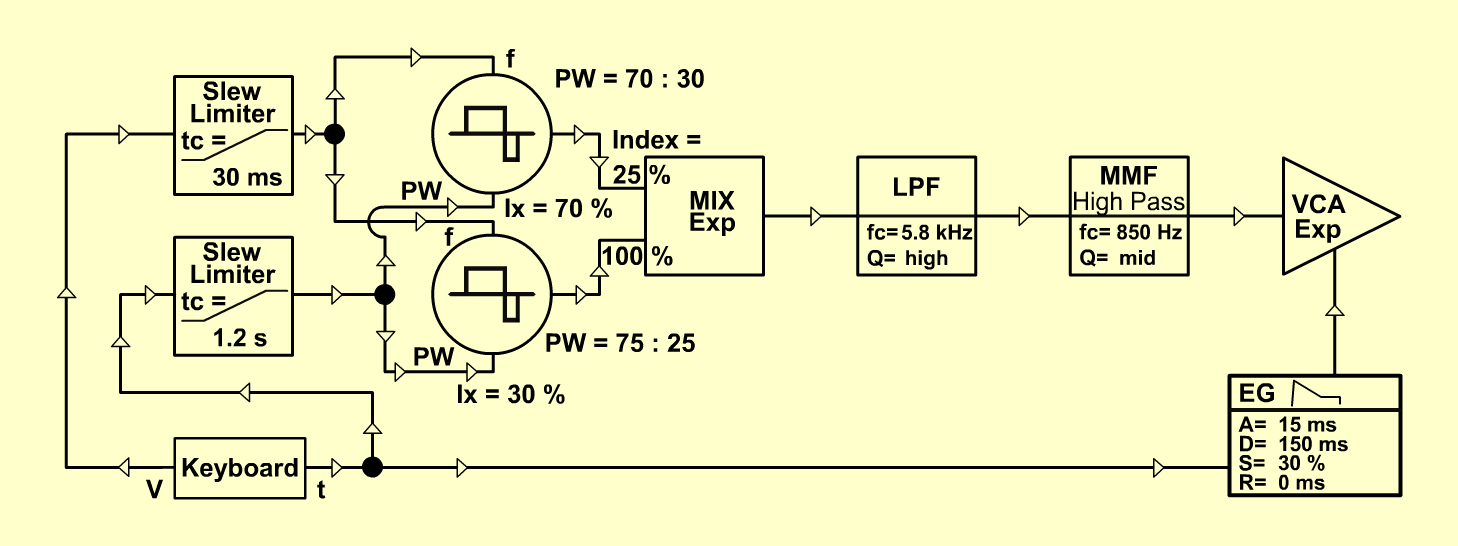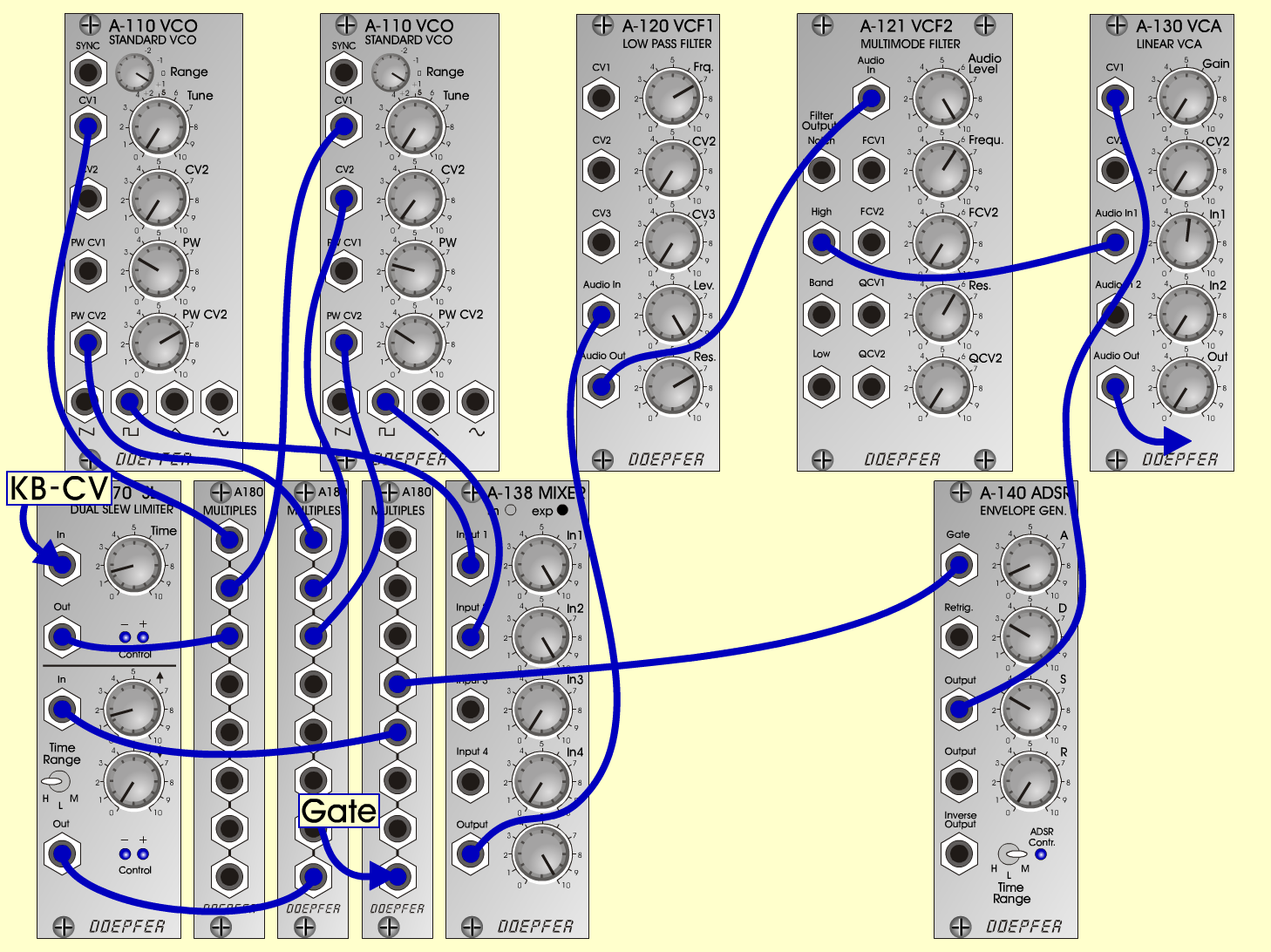V o i c eVoices

 <D o e p f e r A - 100 Connections: Settings: A-110/1 (Pulse)  <=>  A-138exp (Input 1) A-110/2 (Pulse)  <=>  A-138exp (Input 2) A-138exp (Output)  <=>  A-120 (Audio In) A-120 (Audio Out)  <=>  A-121 (Audio In)  A-121 (High)  <=>  A-130 (Audio In) KB (CV)  <=>  A-170top (In) A-170top (Out)  <=>  A-180/1 A-180/1  <=>  A-110/1 (CV 1) A-180/1  <=>  A-110/2 (CV 1) KB (Gate)  <=>  A-180/2 A-180/2  <=>  A-140 (Gate) A-180/2  <=>  A-170bottom (In) A-170bottom (Out)  <=>  A-180/3 A-180/3  <=>  A-110/1 (PW CV 2) A-180/3  <=>  A-110/2 (PW CV 2) A-180/3  <=>  A-110/2 (CV 2) A-140 (Output)  <=>  A-130 (CV 1) A-110/1 (Range = +1, PW = 3, PW CV 2 = 7) A-110/2 (Range = +1, CV 2 = 0.2, PW = 2.5, PW CV 2 = 3.1) A-138exp (In 1 = 10, In 2 = 10, Out = 10) A-120 (Freq = 7, Lev = 10, Res = 7) A-121 (Audio Level = 10, Freq = 6.1, Res = 6) A-170top (Time = 1.5) A-170bottom (up = 1.5, down = 0, Range = H) A-140 (A = 1.2, D = 3, S = 3, R = 0, Range = M) A-130 (Gain = 0, Audio In 1 = 10,  Audio Out = 7.6) VCO tuned to same frequency. 170 Slew limiter (bottom) will offset VCO/2 more and more and let the oscillators beat against each other in a kind of vibrato. Keld SørensenSound samples Voice (261 Hz) Voice (523 Hz) Voice (523 Hz with dig. chorus and reverb)